### SPACE COMMUNICATION CALCULATIONS

INTRODUCTION

In designing systems for space communication it is essential to know the transmitter power that will be required and the antenna sizes, both in space and on the ground, that will allow adequate effective communication with acceptable signal to noise ratio or bit error rate.

Fortunately, communication in space is usually line of sight with no intervening absorption and little scattering media involved. It is therefore easy to calculate and predict the actual communication parameters quite accurately.

THE TRANSMITTING END

The basic physical principle behind all space communication is the inverse square law. This expresses the fact that all electromagnetic radiation spreads out as it propagates and has an intensity that is proportional to the reciprocal (or inverse) of the square of the distance from the source. This is expressed mathematically as:

I = k / r2

where r is the distance from the emitter
and k is the proportionality constant

This law is illustrated graphically below.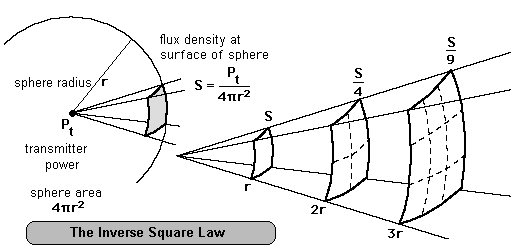At a distance r the beam passes through an area A. At a distance of 2r the beam passes through an area of 4A, and at a distance of 3r the beam passe through an area of 9A. It can be seen that the area increases as the square of the distance. If no absorption of the beam energy occurs, the same power must pass through each area. At a distance of 2r each square of area only receives one quarter the total beam power, and at a distance of 3r each unit of area only receives one ninth of the total beam power. Thus the flux density of the signal (ie the power per unit area) is proportional to the reciprocal square of the distance.

How do we determine the proportionality constant? Imagine we have an isotropic radiator, a transmitter that broadcasts a power of Pt uniformally in all directions. If we also imagine a sphere surrounding the transmitter, with the transmitter at its centre, then the power at each point on the sphere will be the same. Now we know that the total surface area of a sphere of radius r is 4 π r2. All the transmitted power must pass through this area, and so the flux density of the signal at the surface of the sphere is

S = Pt / ( 4 π r2 )
This is also shown in the figure below.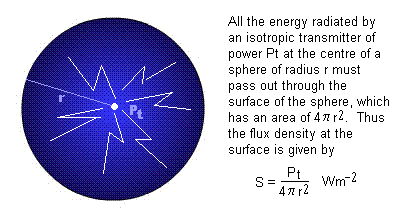Note: If we consider the matter closely, we see that the inverse square law is a consequence of us living in a three dimensional universe. In a 2D universe we would have an inverse distance law where S2D = Pt / ( 2 π r ). That is, in 2D the power falls off as the inverse of the distance, not the inverse square of the distance (this means that the power falls off at a less rapid rate).

Very few transmitting system are isotropic radiators. Normally they send out signals in a beam, which concentrates power along the boresight of the antenna at the expense of reducing the signal in other directions. The beam has a beamwidth, which may be broad (if the antenna gain is low) or narrow (when the antenna gain is high).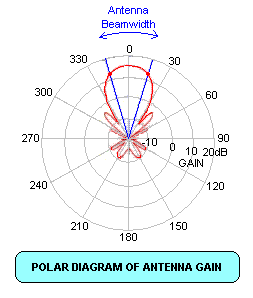Non-isotropic radiators still obey the inverse square law. Even laser beams, which have extremely narrow beams, obey the inverse square law. No device has yet been made whose radiated energy does not diverge with distance from the emitter. For a non-isotropic emitter with an antenna gain Gt we use the 'effective' radiated power (the power at the centre of the beam) where:

Peff = Gt Pt , and thus

S = Gt Pt / ( 4 π r2 )

THE RECEIVING END

 At the receiving end is an antenna that intercepts a portion of the transmitted power. The main parameter associated with a receiving antenna is its effective area. For a parabolic antenna, as shown in the diagram, the effective area is a fraction 'e' of the physical cross section that the antenna presents to the incoming signal. That fraction 'e' is thus termed the efficiency of the antenna. For a different type of antenna, the effective area can be determined as described later.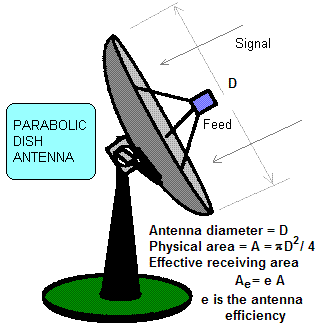Now the units of flux density are watts per square metre (W m-2) and the units of area are square metres. The power (in watts) collected by the antenna is the thus the flux density times the effective collecting area of the antenna:

Pr = S Ae
Substituting for S and Ae we find that:
Pr = e D2 Peff / ( 16 r2 )
Now in any receiving system this signal power has to contend with a noise power. This noise can arise from external sources but it is also unavoidably generated in the receiver by normal electronic processes associated with active devices such a transistors that are necessary to amplify the signal. Even resistors generate noise, and this noise is proportional to the temperature of the resistor. It is thus usual to talk about the noise temperature of a receiver as the temperature of a matched resistor connected to an ideal (no-noise) receiver that generates the same noise power output as the actual receiver.

This noise power is given mathematically by the formula:

Pn = k B T
where
k is a constant (called Boltzmann's constant)
B is the bandwidth of the receiver (in Hz), and
T is the equivalent noise temperature of the receiving system
In SI units, k has the value 1.38 x 10-23.

For more information on communication noise refer to the ASA article on noise.

For a signal to be detectable it must be greater than some number 'n' times the noise. That is:

Pr > n Pn
The value n = Pr / Pn is usually termed the signal to noise ratio or SNR (although an alternative definition of SNR is SNR = (Pr + Pn) / Pn ). We will use the first definition. SNR is often expressed in decibels. Thus n=2 implies SNR=3dB, n=10 implies SNR=10 dB and n=100 implies SNR=20dB.

For voice communication the SNR must be greater than 3 dB if the signal is to be understood at all. A value of 10 dB is normally the minimum desirable SNR and an SNR of 20dB (n=100) is required for perceived 'noise-free' communication. An even higher SNR might be required for a noise free TV image. However, for some types of modulation, in particular that known as 'spread-spectrum' modulation it is still possible to decode a signal for values of n less than 1 (ie negative values of the SNR in decibels).

SNR is calculated from the expression:

n = Pr / Pn = e D2 Gt Pt / ( 16 r2 k B T )
If the collecting area of an antenna needs to be calculated it can be done using what is known as the reciprocity theorem. This states that the gain G of a receiving antenna is identical to the gain of the same antenna used to transmit a signal. This transmitting gain can be measured on an 'antenna range' by comparison with an omnidirectional antenna (or one with a known gain). The effective collecting area of the antenna is then calculated from:
Ae = λ2 G / ( 4 π )
or
e D2 = λ2 G / π2
We now have enough information to calculate communication parameters for a wide range of space communication situations.

Note: Engineers often use 'logarithmic' versions of these formula so that quantities can be added and subtracted instead of multiplied and divided. With computers this is no longer really necessary. Many engineering textbooks on communication also give an expression for "free-space" path loss in decibels which depends on frequency. This is entirely misleading, as we have proven above that free space path loss is entirely due to the geometry of space and is independent of wavelength or frequency. The reason the engineers get confused is because they use the wrong parameter for the receiving antenna. They use gain instead of collecting area, and in the process introduce a wavelength dependence of the gain, which they then wrongly ascribe to the free-space path loss. These equations work, but give a misleading picture of the physical situation.For some of the following calculations we need to consider the beamwidth of the antenna. For an antenna with an effective linear aperture 'a' the half-power beamwidth is given by the expression:

θ = λ / a
where
θ is the beamwidth in radians
λ is the operating wavelength in metres
a is the aperture in metres
Note that λ(m) = 300 / freq(MHz)

For a parabolic dish antenna a = D (the dish diameter). For other types of antenna we can compute the effective diameter through the gain equation.

The following two graphs plot antenna beamwidth as a function of antenna diameter.The following sections investigate the transmitting powers and antenna sizes necessary to give specified SNR's over various distances.

COMMUNICATING WITH THE INTERNATIONAL SPACE STATION

The International Space Station (ISS) hosts an amateur radio experiment (ARISS) whereby schools and amateur radio operators can communicate with astronauts. The ISS orbits the Earth at an altitude of around 400 km. This is toward the lower end of the range of orbits called Low Earth Orbit (LEO). At this altitude atmospheric drag is significant and the ISS orbit must be periodically boosted to keep it from reentering back to Earth. Thus the orbital altitude may change from about 350 to 450 km.

One of the problems with communications from Earth to LEO is that the satellite moves quickly, and that the distance to the satellite changes by a large factor from when it is overhead to when it is on the horizon - see diagram below: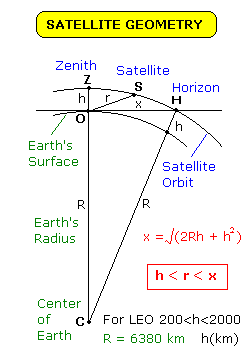Thus the range to the ISS may vary from ~400 km for an overhead pass to almost 2300 km when the station is on the horizon - either at AOS (acquisition of signal) or LOS (loss of signal). Since received power varies as the square of the distance, this makes for a 33 times (15 dB) change in signal strength.

The rapid change in direction to the ISS as it passes across the communicator's sky could be a problem for low cost communication if a directional antenna was required. This is because the directional antenna would have to track the ISS as it moved. Fortunately the low frequency used (145.825 MHz) allows the use of hand-held radios with almost omnidirectional whip antennae.

Our problem for computation will thus be to determine the received SNR for the range of distances over which communication must take place. We will do so for three transmit powers, 1 W, 3 W and 5 W, which are typical of the powers that simple amateur hand-held transceivers might employ. These are effective radiated powers which might result from a transmit power half of that times an antenna gain of two. We also assume a clear line of sight between ground communicator and the ISS. The results are shown in the graph below: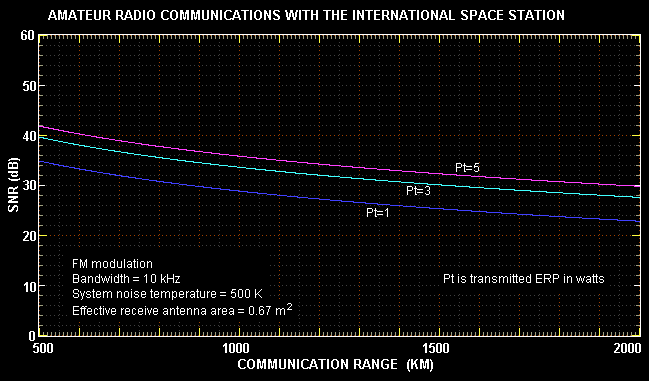As can be seen this results in a strong signal for the whole pass of the ISS over the ground station. A 20 dB SNR using narrow-band FM modulation is sufficient to ensure no-noise ('full-quieting') communication.

LOW EARTH ORBIT COMMUNICATIONS

A more typical LEO satellite might orbit at around 800 km altitude. From the above table this will result in ranges out to over 3000 km during the ground station pass.

Let us consider the case of a polar orbiting weather satellite at 800 km altitude and transmitting low resolution imagery at VHF (137 MHz) and high resolution imagery at L-band (1700 MHz). We will assume each transmitter radiates with an effective power of 5 watts. For the receiver we will need a bandwidth of 70 kHz and a system noise temperature of 300 K.

For this exercise will look at the SNR that results from the use of 3 different receive antenna gains. One at 3 dB which is what we expect from a non-tracking omni-directional antenna, 13 dB which is the typical gain of a 10 element yagi antenna, and 27 dB gain antenna. The latter can be achieved at L-band with a 1.5 m dish, whereas it would require a much larger dish to give this gain at VHF - or alternatively an enormous array of yagis.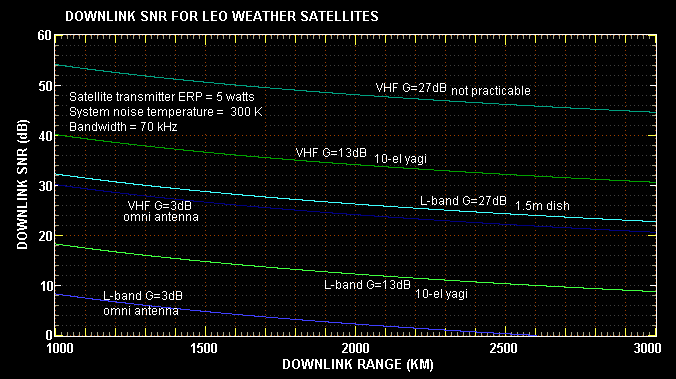We immediately see that a non-tracking antenna can be used at the VHF frequency but not at L-band. We really need the 27 dB (1.5 m dish) at L-band to get a good quality signal. This means that L-band and above requires a tracking antenna, with all the additional hardware and expense this entails.

In fact, the situation is even worse at L-band than the above figure depicts and that is because we need a bandwidth considerably more than 70 kHz to download the high resolution imagery. This decreases the SNR. To offset this, the satellite L-band transmitter radiates substantially more power than does the VHF one. We are also helped by nature in that cosmic noise, which sets our system noise at VHF decreases rapidly with frequency and allows the use of a lower system noise temperature at L-band.

COMMUNICATING TO GEOSYNCHRONOUS ORBIT

Geosynchronous satellites orbit at an altitude of 36,000 km. This large altitude means we always need a directional antenna for geosat communications. However, we have two points in out favour. Because the orbit is at a radius 6.6 times larger than the Earth's radius, the variation in range from the zenith to the horizon is not very great (about 17%). We can assume a mean range of 38,000 km in our calculations. The second benefit of the geosynchronous orbit is that such a satellite stays relatively much in the same place in the sky and thus it does not need to be tracked. This makes possible direct to home TV broadcasts at low cost (because the antenna can be fixed in look position).

Geosynchronous downlinks vary in power and bandwidth. Bandwidths can be very narrow in the case of beacons to very large in the case of TV transmissions which might be 36 MHz wide. Powers can vary from a few watts to hundreds of watts. In the graph below we will look a how SNR varies with transmission power (ERP) and bandwidth. We will assume a low noise receiving system noise temperature of 50 K.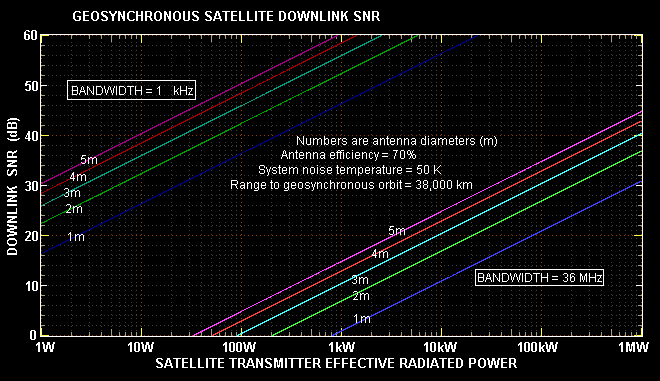LUNAR COMMUNICATIONS

Communication with the Moon is simplified in that the distance from the Earth to the Moon is effectively constant, no matter where the Moon is in the sky. In the example below we are interested in the terrestrial antenna size we would need to receive data from a scientific package left on the lunar surface together with a one watt transmitter with a non-directional antenna.

Although the Earth always appears at the same point in the sky from a fixed position on the Moon, and thus a high-gain antenna antenna pointed to the Earth does not have to track, it must be pointed in the right direction in the first place. This might not be a problem for a sophisticated 'lander' but it might impose an impossible weight penalty on a simple package.We can see that even for a low data rate (1 kHz bandwidth) we need at least a 15 m dish to get an acceptable SNR (10 dB). Real-time TV (with a required bandwidth in excess of 100 kHz) is simply not practicable in this situation, even with very large antenna.

COMMUNICATING FROM A HELIOCENTRIC ORBIT

A heliocentric orbit is one that revolves around the Sun. Any spaceprobe sent to explore another planet must first be put into a heliocentric transfer orbit before it is then inserted into the planetocentric orbit. Communication with a spacecraft in any heliocentric orbit naturally involves much larger distances than for any orbit around the Earth.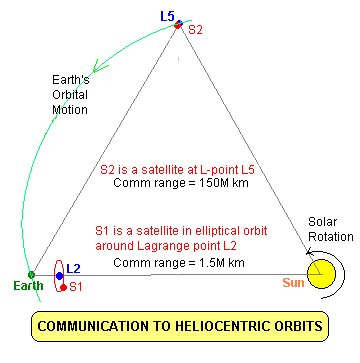There are special gravitationally stable points around any two body system (eg Earth-Sun). Earth's Lagrange points have special significance for satellites. The L2 point allows unobstructed continuous solar observation, and also enables early detection of solar originated space weather before it hits the Earth (eg solar wind shocks and CMEs). The L5 is useful because it can see 'behind' the Sun and give warning of large active regions and sunspots before they rotate into Earth's view.

Communicating to and from L5 involves no special problems save for the large distance. However, despite the small distance, receiving data from L2 is a big problem. If your antenna is pointed at L2 it is also pointed at the Sun, and the very high temperature of the Sun (it can be up to a million Kelvin at VHF wavelengths) determines the noise temperature of your receiving system. It is no use having a 50 K low noise amplifier if the Sun injects 50,000 K of noise into the system.

For this reason no satellite is ever put directly at the L2 point. Instead it is placed into an elliptical orbit some 100,000 km in radius around the L2 point. The plane of the orbit is perpendicular to a line from the Earth to the Sun. In this way the receive antenna on Earth will never have to point at the Sun. From the geometry of the situation we can determine that such a satellite will be approximately 100,000/1,500,000 radians from the Sun. This is just under 4 degrees. Our antenna should probably have a beamwidth no more than 1 degree to ensure that it avoids the hot corona of the Sun that extends quite a distance above the apparent surface. From the above table we see that at S-band we will need a 7 to 8 metre dish to achieve this.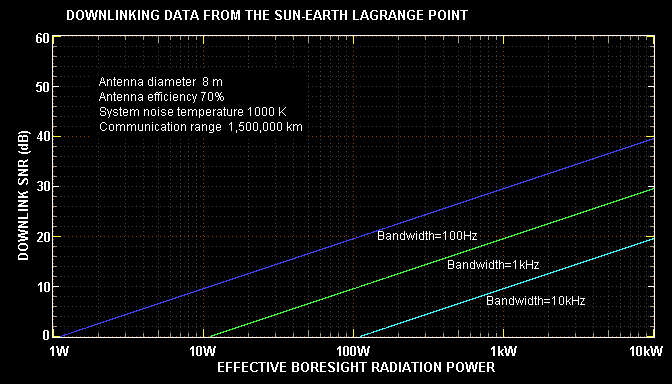For communicating to L5 and interplanetary distances only the largest antennae can provide an acceptable signal to noise ratio. As the background sky is quite cold, very low noise amplifiers can be used to give a very low system noise temperature.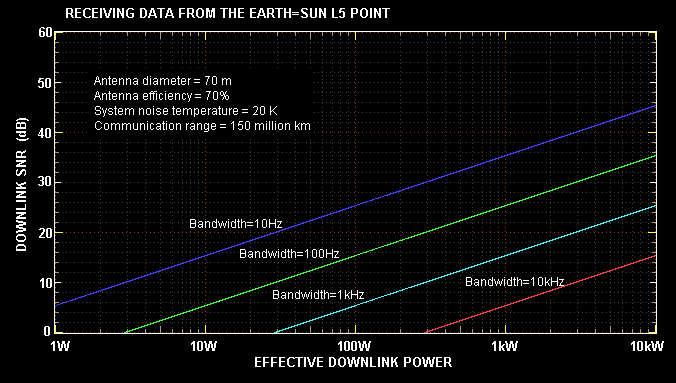COMMUNICATING WITH MARS

 The problem with communication to the planets is that they are very far away, their distance from the Earth varies enormously as they and the Earth orbit around the Sun at different speeds, and they go near to and behind the Sun every so often, which makes communication impossible. Distances are measured in AU (Astronomical Units) which is just under 150 million km. Earth orbits the Sun between 0.98 (perihelion) and 1.02 (aphelion) AU. Mars orbits with a perihelion of 1.38 and aphelion of 1.67. (Mars has a more eccentric orbit than the Earth). This means that the absolute minimum and maximum distances between Mars and the Earth are 0.36 and 2.69 AU. Giving consideration to the orbits and the fact that communication is not possible at the absolute maximum, a more typical communication range variation is from 0.5 to 2.5 AU. This is a 5 times variation which results in a 14 dB change in the signal to noise ratio.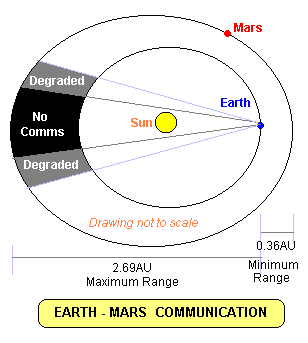As for the case for L5 communication, only the largest antennae and the lowest noise amplifiers can provide a useful SNR to download digital data at a reasonable rate. The uplink side will always have a much better SNR at the spacecraft because of the much larger transmitter power available from an Earth based communication station.The QBASIC program COMMPARS.BAS allows an unknown communication variable to be calculated as long as all the other required communication parameters are specified. The program allows alternate inputs (eg antenna collecting area as either dish diameter or antenna gain either in dB or as a number). Because of this it takes a while to learn what set of parameters must be specified. If a parameter or its alternates are left blank (CR on input) then that is the parameter that will be calculated. There are no error traps, so that if more than one required input/alternate is not specified the program may crash. This program is presented as a learning tool only.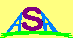Australian Space Academy